Home > INT3 > Chapter 11 > Lesson 11.1.3 > Problem11-32

11-32.
1. Use your understanding of adding fractions to add the rational expressions below. Then simplify your solutions, if possible. Homework Help ✎

1.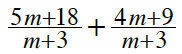2.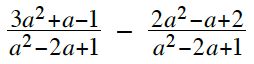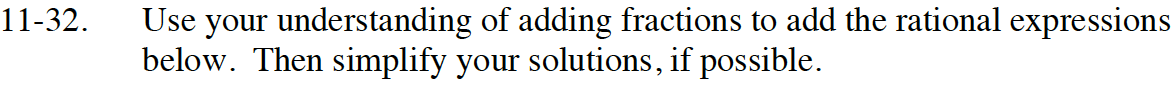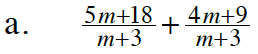Because the denominators are the same, you can add the numerators together.

Factor 9 out of the numerator and simplify the equation by looking for factors that make 1.

9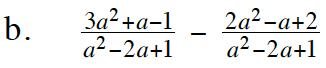Subtract the numerators, then try factoring.
Be sure to distribute the negative when performing the subtraction.

$\frac{(3\textit{a}^2+\textit{a}-1)-(2\textit{a}^2-\textit{a}+2)}{\textit{a}^2-2\textit{a}+1}$

Look for factors that make 1.